Question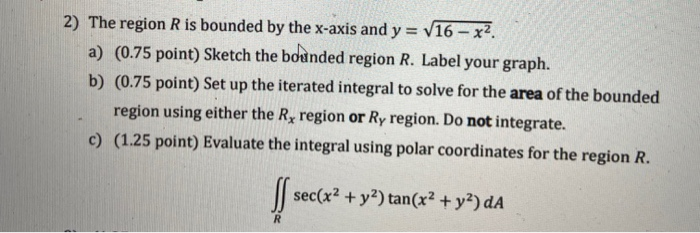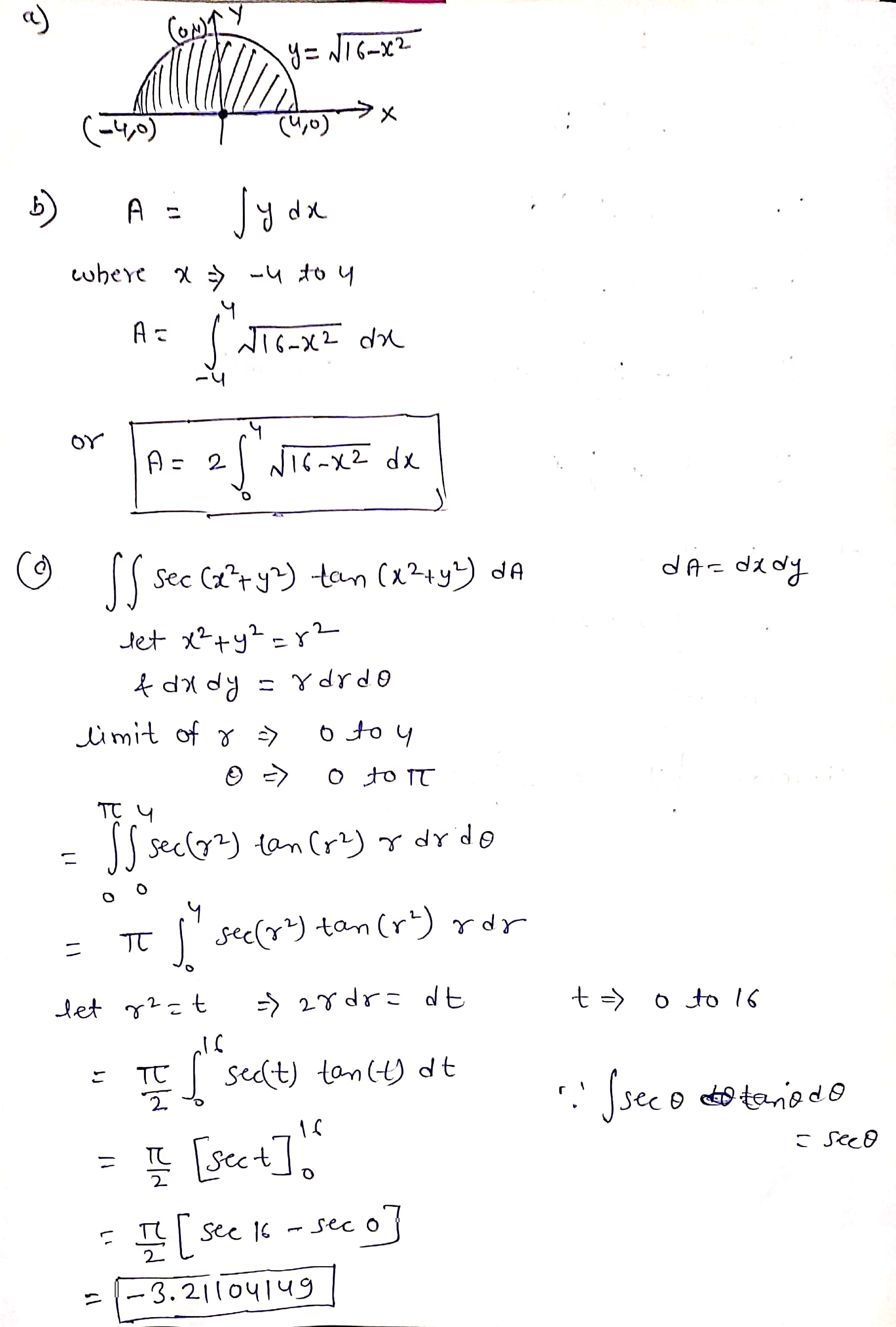#### Earn Coins

Coins can be redeemed for fabulous gifts.

Similar Homework Help Questions
• ### 2) The region R is bounded by the x-axis and y = V16 - x2 a)...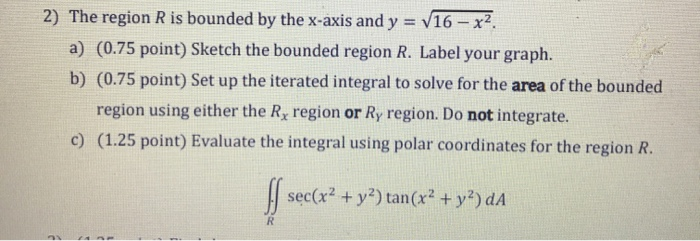2) The region R is bounded by the x-axis and y = V16 - x2 a) (0.75 point) Sketch the bounded region R. Label your graph. b) (0.75 point) Set up the iterated integral to solve for the area of the bounded region using either the Rx region or Ry region. Do not integrate. c) (1.25 point) Evaluate the integral using polar coordinates for the region R. sec(x2 + y2) tan(x2 + y2) dA R

• ### 2) The region R is bounded by the x-axis and y = V16 – x2. a)...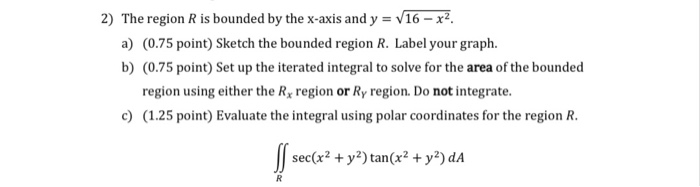2) The region R is bounded by the x-axis and y = V16 – x2. a) (0.75 point) Sketch the bounded region R. Label your graph. b) (0.75 point) Set up the iterated integral to solve for the area of the bounded region using either the Rx region or Ry region. Do not integrate. c) (1.25 point) Evaluate the integral using polar coordinates for the region R. S sec(x2 + y2) tan(x2 + y2) da R

• ### 2) The region R is bounded by the x-axis and y = V16 – x2 a)...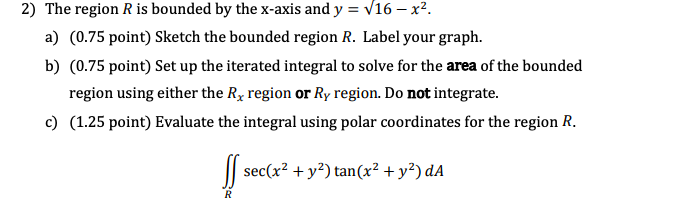2) The region R is bounded by the x-axis and y = V16 – x2 a) (0.75 point) Sketch the bounded region R. Label your graph. b) (0.75 point) Set up the iterated integral to solve for the area of the bounded region using either the Rx region or Ry region. Do not integrate. c) (1.25 point) Evaluate the integral using polar coordinates for the region R. S sec(x2 + y2) tan(x2 + y2) da R

• ### The region R is bounded by the x-axis and y = V16 – x2 a) Sketch...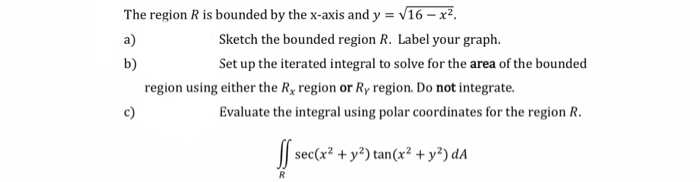The region R is bounded by the x-axis and y = V16 – x2 a) Sketch the bounded region R. Label your graph. b) Set up the iterated integral to solve for the area of the bounded region using either the Rx region or Ry region. Do not integrate. Evaluate the integral using polar coordinates for the region R. sec(x2 + y2) tan(x2 + y2) da c) R

• ### Show all of your work. Label the axes and scale on your graph. The sketch should...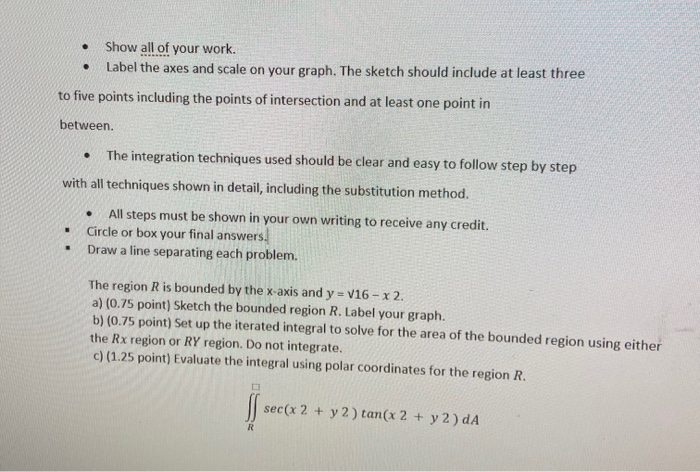Show all of your work. Label the axes and scale on your graph. The sketch should include at least three . to five points including the points of intersection and at least one point in between The integration techniques used should be clear and easy to follow step by step with all techniques shown in detail, including the substitution method. . . All steps must be shown in your own writing to receive any credit. Circle or box your final...

• ### 6. (4 pts) Consider the double integral∫R(x2+y)dA=∫10∫y−y(x2+y)dxdy+∫√21∫√2−y2−√2−y2(x2+y)dxdy.(a) Sketch the region of integration R in Figure 3.(b)...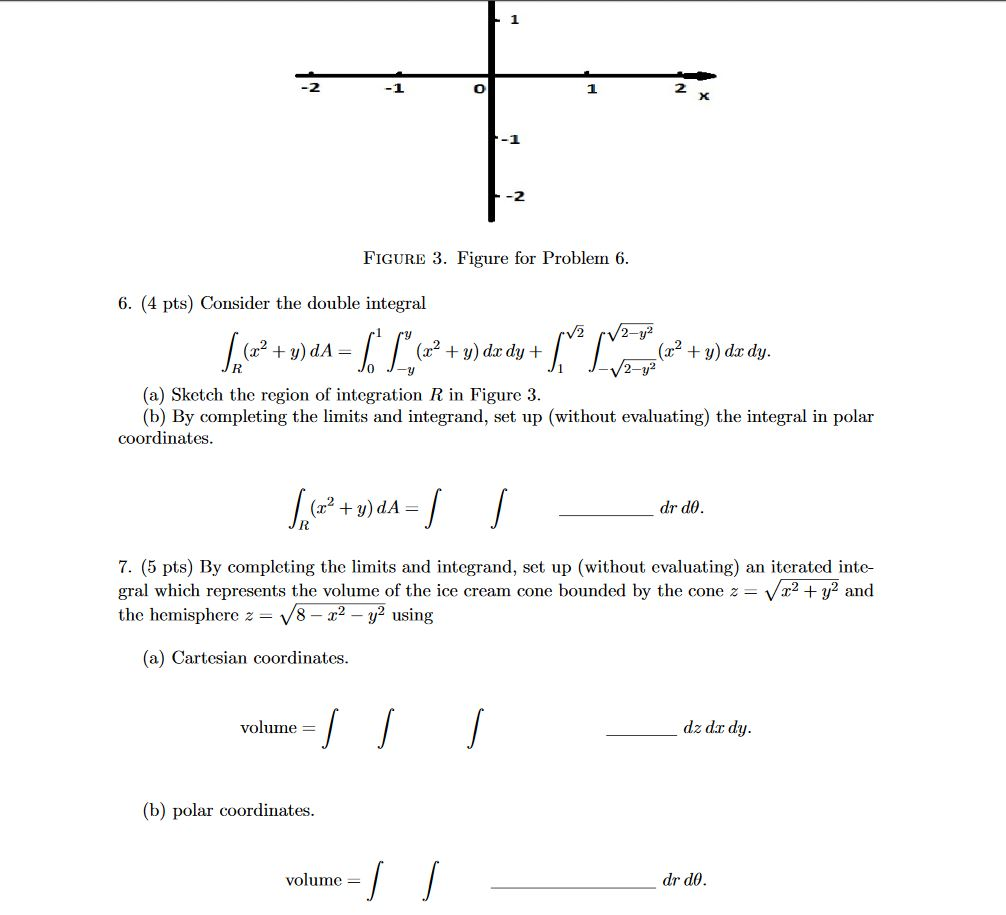6. (4 pts) Consider the double integral∫R(x2+y)dA=∫10∫y−y(x2+y)dxdy+∫√21∫√2−y2−√2−y2(x2+y)dxdy.(a) Sketch the region of integration R in Figure 3.(b) By completing the limits and integrand, set up (without evaluating) the integral in polar coordinates.∫R(x2+y)dA=∫∫drdθ.7. (5 pts) By completing the limits and integrand, set up (without evaluating) an iterated inte-gral which represents the volume of the ice cream cone bounded by the cone z=√x2+y2andthe hemisphere z=√8−x2−y2using(a) Cartesian coordinates.volume =∫∫∫dz dxdy.(b) polar coordinates.volume =∫∫drdθ. -1 -2 FIGURE 3. Figure for Problem 6. 6. (4 pts)...

• ### 6. (4 pts) Consider the double integral∫R(x2+y)dA=∫10∫y−y(x2+y)dxdy+∫√21∫√2−y2−√2−y2(x2+y)dxdy.(a) Sketch the region of integrationRin Figure 3.(b) By completing...6. (4 pts) Consider the double integral∫R(x2+y)dA=∫10∫y−y(x2+y)dxdy+∫√21∫√2−y2−√2−y2(x2+y)dxdy.(a) Sketch the region of integrationRin Figure 3.(b) By completing the limits and integrand, set up (without evaluating) the integral in polar coordinates. -1 -2 FIGURE 3. Figure for Problem 6. 6. (4 pts) Consider the double integral V2 /2-y² + = (x2 + y) dx dy + + y) do dy. 2-y2 (a) Sketch the region of integration R in Figure 3. (b) By completing the limits and integrand, set up (without evaluating)...

• ### 1) Given the following iterated integral. ex/Y DA R = y = 4x, y = -xy...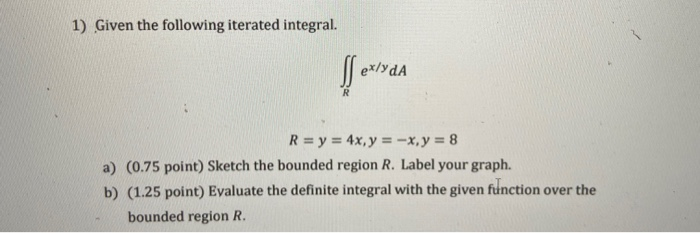1) Given the following iterated integral. ex/Y DA R = y = 4x, y = -xy = 8 a) (0.75 point) Sketch the bounded region R. Label your graph. b) (1.25 point) Evaluate the definite integral with the given function over the bounded region R.

• ### 1) Given the following iterated integral. ex/ydA R = y = 4x, y = -x, y...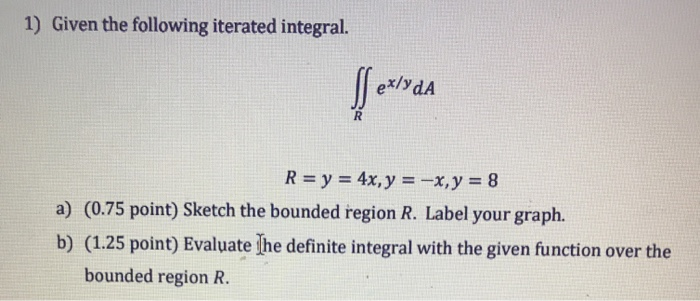1) Given the following iterated integral. ex/ydA R = y = 4x, y = -x, y = 8 a) (0.75 point) Sketch the bounded region R. Label your graph. b) (1.25 point) Evaluate The definite integral with the given function over the bounded region R.

• ### 1) Given the following iterated integral. ex/ydA R= y = 4x, y = -x,y = 8...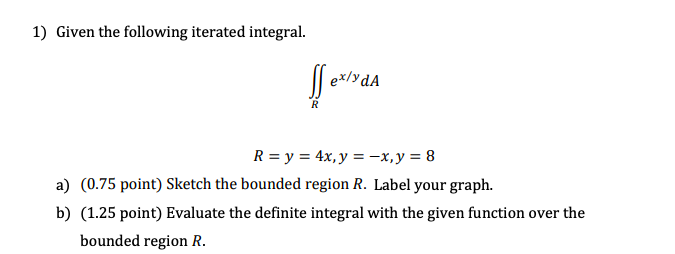1) Given the following iterated integral. ex/ydA R= y = 4x, y = -x,y = 8 a) (0.75 point) Sketch the bounded region R. Label your graph. b) (1.25 point) Evaluate the definite integral with the given function over the bounded region R.